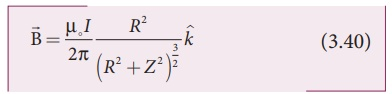Home | | Physics 12th Std | Magnetic field produced along the axis of the current carrying circular coil

# Magnetic field produced along the axis of the current carrying circular coil

Biot - Savart Law: Magnetic field produced along the axis of the current carrying circular coil

Magnetic field produced along the axis of the current carrying circular coil

Consider a current carrying circular loop of radius R and let I be the current flowing through the wire in the direction as shown in Figure 3.40. The magnetic field at a point P on the axis of the circular coil at a distance from its center of the coil O. It is computed by taking two diametrically opposite line elements of the coil each of lengthat C and D. Letbe the vector joining the current element (I) at C to the point P.According to Biot-Savart’s law, the magnetic field at P due to the current element IisThe magnitude of magnetic field due to current element Iat C and D are equal because of equal distance from the coil. The magnetic fielddue to each current element Iis resolved into two components; dB sin θ along y - direction and dB cos θ along z - direction. Horizontal components of each current element cancels out while the vertical components (dB cos θ) alone contribute to total magnetic field at the point P.

If we integratearound the loop,sweeps out a cone as shown in Figure 3.40, then the net magnetic fieldat point P isusing Pythagorous  theorem r2= R2+Z2 and integrating line element from 0 to 2πR, we getNote that the magnetic fieldpoints along the direction from the point O to P. Suppose if the current flows in clockwise direction, then magnetic field points in the direction from the point P to O.

EXAMPLE 3.17

What is the magnetic field at the center of the loop shown in figure?Solution

The magnetic field due to current in the upper hemisphere and lower hemisphere of the circular coil are equal in magnitude but opposite in direction. Hence, the net magnetic field at the center of the loop (at point O) is zero.

Tags : Biot - Savart Law | Physics , 12th Physics : Magnetism and Magnetic Effects of Electric Current
Study Material, Lecturing Notes, Assignment, Reference, Wiki description explanation, brief detail
12th Physics : Magnetism and Magnetic Effects of Electric Current : Magnetic field produced along the axis of the current carrying circular coil | Biot - Savart Law | Physics